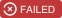# Factor numbers using a quantum computerdwave-examples, updated 🕥 2022-01-21 22:52:16# Factoring

This code demonstrates the use of the D-Wave system to solve a factoring problem. This is done by turning the problem into a three-bit multiplier circuit.

## Usage

A minimal working example using the main interface function can be seen by running:

```bash python demo.py```

The user is prompted to enter a six-bit integer: P, which represents a product to be factored.

```bash Input product ( 0 <= P <= 63):```

The algorithm returns possible A and B values, which are the inputs the circuit multiplies to calculate the product, P.

## Code Overview

Integer factoring is the decomposition of an integer into factors that, when multiplied together, give the original number. For example, the factors of 15 are 3 and 5.

D-Wave quantum computers allow us to factor numbers in an entirely new way, by turning a multiplication circuit into a constraint satisfaction problem that allows the quantum computer to compute inputs from a predefined output. Essentially, this means running the multiplication circuit in reverse!

A Boolean logic circuit is usually viewed as computing outputs from inputs based on the logic of the gates. However, the problem can also be thought of as seeking an assignment of values to the inputs and outputs consistent with the logic of all the gates in the circuit. This perspective of constraint satisfaction has no directionality. That is, input values do not need to flow through a series of gates to yield a result, as they do in a multiplication circuit.

## Issues

### Demo on any advantage of D-Wave over a PC

opened on 2022-01-22 09:43:21 by Mather10

Could you please demonstrate any advantage of using D-Wave to factor a number over using a PC to do that? Thanks.

## Releases

### 0.0.3 2018-04-11 23:56:35

#####D-Wave Systems Examples

D-Wave Ocean code examples

constraint-satisfaction-problem intelligence intermediate